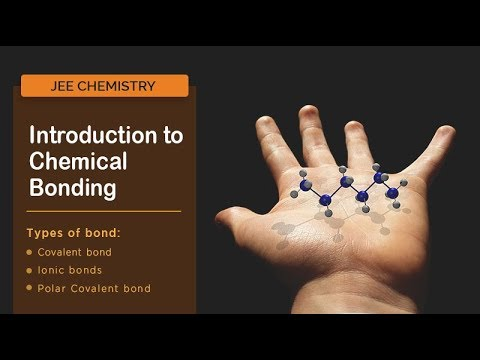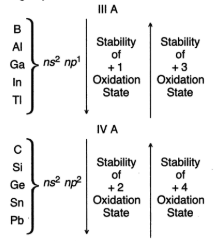# Variable Valency

## What is Valency?

Valency is the combining capacity of an element. The number of electrons donated or accepted by an atom of an element so as to have electronic configuration of nearest noble gas is called its valency.

## What is Variable Valency?

Sometimes, because of different conditions under which a chemical reaction takes place, the valency of the same element changes. Thus metals like lead, tin, copper, mercury, iron etc exhibit variable valency.

Normally, the metals donate electrons from their valence shell, so as to form positively charged ions. However, some metals lose electrons from the shell next to the valence shell. In such a situation the element exhibits more than one electropositive valency. Such elements are said to have variable valency.

## Recommended Videos## Variable Electrovalency

The electrovalency of an element is equal to the number of electrons lost or gained by its atom during the formation of ionic bonds. The s-block elements do not show variable valency. However, p-block elements with higher atomic number transition and inner transition elements show variable valency.

There are two main reasons for showing variable valency

1. Inert pair effect in p-block elements.
2. Small energy difference between ns and (n-1)d sub shells in transition elements and ns and (n-2) sub shells in inner transition elements.

### 1. Inert Pair Effect

The electronic configurations of III A and IV A group elements are asThe elements of III A group show +3 oxidation state mainly. But as we go down the group the oxidation state +1 becomes more and more pronounced. Similarly the elements of IV A group show +4 oxidation state in general but as we go down the group the +2 state becomes more and more pronounced.

The two s electrons (ns2) in the valence shell tend to remain inert and do not participate in formation of bonds. This is called inert pair effect. Thus the inert pair effect is the main cause of variable valency in p block elements.

### 2. Energy Difference between Orbitals

In transition elements the variation in oxidation state is due to involvement of incomplete d-orbitals in addition to electrons of ns orbital. So the electrons from both the energy levels can be used for bonding.

Similarly, inner transition elements show variable valency due to incomplete filling of f-orbitals. So in addition to ns electrons, the electrons from f-orbitals may be used for chemical bond formation.

## Variable Valency Examples

Certain elements have more than one valency. They exhibit variable valency. For example, copper, tin, iron and mercury exhibit variable valency. Copper combines with oxygen to form cuprous oxide (Cu2O) and cupric oxide (CuO).

Valency of oxygen being two, combining capacity of copper in the first case is one and that in the second case is two. The suffix “ous” is used to indicate the lower valency and the suffix “ic” to indicate the higher valency. The following table gives the names of some compounds with variable valencies of elements.

 Compound Valency Ion Cuprous (Cu2O) 1 Cu+ Cupric oxide (CuO) 2 Cu2+ Ferrous oxide (FeO) 2 Fe2+ Ferric oxide (Fe2O3) 3 Fe3+
 Element Valency Ion Mercury (Hg) 1 Mercurous (Hg+) 2 Mercuric (Hg2+) Tin (Sn) 2 Stannous (Sn2+) 4 Stannic (Sn4+)

Another example is iron has two electrons in its valence shell. On donating these electrons, its valency is +2. However, under specific experimental conditions, it can lose another electron from the next valence shell. In such a situation the ion formed has +3 electropositive valency.

## Determination of Valencies of Elements from the Formula of Compounds

We know that the valency of chlorine (Cl) is 1.

• In sodium chloride (NaCl), the valency of sodium (Na) is 1.
• In calcium chloride (CaCl2), the valency of calcium (Ca) is 2.
• In ferric chloride (FeCl3), the valency of iron (Fe) is 3.
• In phosphorus trichloride (PCl3), the valency of phosphorus (P) is 3.

The above example suggests a simple rule of determining the valency of elements in the compounds having two elements.

The number of units of valency of all the atoms of one element is equal to the number of units of valency of all the atoms of the other elements.

## Solved Example

Find the valency of magnesium (Mn) in the compound Mn2O7

Solution:

No of valency units of 2 atoms of manganese (Mn) = No of valency units of 7 atoms of oxygen.

= 7 x 2

= 14 valency units

Therefore, No of valency units of one atom of Mn = 14/2 = 7

So the Valency of Mn in Mn2O7 is 7.

## Frequently Asked Questions on Variable Valency

Q1

### What is Valency and variable valency?

The valence is known as an element’s combining power. Some elements vary in their ability to interact with other elements, depending on the nature of the reaction; variable valence is called this property. Iron (Fe), for example, may have a valence of both 2 and 3.

Q2

### What is the cause of variable valency?

The electrons which are present in an atom’s outermost shell are called valence electrons. So one or more electrons from the core’s outermost shell may also be lost after the removal of valence electrons. Such elements therefore indicate two or more different valencies i.e. they have variable valentia.

Q3

### What is a variable valency example?

Some elements join with other atoms to give, accept or exchange electrons in different amounts depending on the essence of the reaction. For starters, iron combines with oxygen to form both ferrous oxide and ferric oxide. It is called valence element.

Q4

### What is the Valency of oxygen?

Oxygen has a valence value of 2. Since it is 2,6, it only requires 2 more electrons to complete it is an octet. Even for elements with n= 1,2,3 and 4 electrons in the outer shell the valence is equal to n but for elements with n= 5,6 and 7 electrons in the outer shell the valence is equal to 8-n.

Q5

### Why does phosphorus show variable valency?

Since the phosphorus valence is 3 but due to the vacant d orbital they will increase their valence. It should be 3 since the valence shell of phosphorus contains 5 electrons. Therefore it accepts 3 electrons and completes its bit, since it will be much simpler to accept 3 electrons than to give 5 electrons.

Test Your Knowledge On Variable Valency!